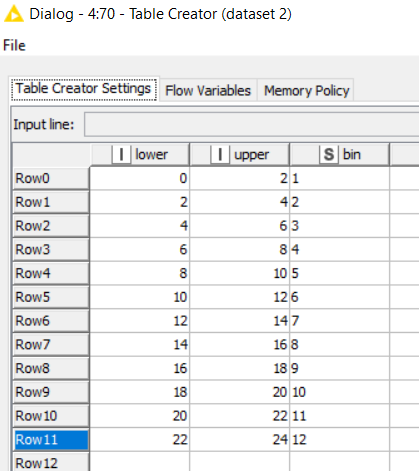# Group / Shrink time series data

Hi all,
I have a big table of measurements taken every second. Now I want to analyze trend and max/min values in a plot graph. The table size is far too big for the line plot node so I decided to kind of group the time series to intervals of let’s say every 2 hours.
For each of these intervals I would calculate average, min/max and maybe other trend values over all the measurements of the specific interval. This should be done in a loop over the complete big dataset.

The trend and min/max values of each interval should later be in a new table with then drastically reduced size. This new table can then be shown with the line plot node.

Would that be possible and how do I configure this process? Any idea?

Hi @Juppes

A possible solution could be to use the -Moving Aggregation- node where you could do convolution filtering based on an odd size sliding window of your choice (here in image is 21) and with the convolution operator of your choice too (here is mean but it could be max, min, median, etc.)Once you have calculated the convolutional operation of your choice based on a sliding window, you need to do a linear sampling of your data to reduce it to the size you want. Here it should be 1/21, so you need to configure a -Row Sampling- node as follows:

I’m setting here to “5%” the linear sampling since it corresponds to roughly 1/21 row sampling.

Doing this way one should be able to obtain a “shrinked” dataset (undersampled by 1/21).

Hope this trick helpsBest

Ael

3 Likes

Hi @Juppes ,
another trick could be

• extract hours with the “Extract Date & Time Fields” node
• create a table like thisand use it as a dictionary for the “Binner (Dictionary)” node. This way each row is assigned to a time bin
• “Group by” the bin label and calculate aggregation functions

Hope it helps

4 Likes

Thanks @aworker for this great solution! It works very good. Only issue is that it takes some time to finish my dataset of a few millions of records.

1 Like

Thanks also @duristef. Your solution helped a lot to bring me to my final way:
I extracted date&time fields as you mentioned and then simply carried out group by operation on the date & time fields down to the hour column. With that I calculate my aggregation functions over all values of each hour. So I could shrink the amount of data significantly. Thanks again!

1 Like

This topic was automatically closed 90 days after the last reply. New replies are no longer allowed.